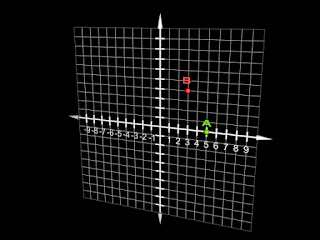### Research: Chapter II

To begin, some background in mathematics must be given and understood. Let us first consider the number line. The number line consists of a series of one-dimensional points representing each real number (rational numbers and irrational numbers). It is widely accepted that points do not have length. If it were not so, then we could find an infinite number of other points between the two "sides" of each point. Thus, it is a contradiction that an object with infinite length (the number line) is made up of an infinite series of points that have no length. However, for the sake of geometrical arguments, we have defined a line as it is above. The number line is represented below:A point limited to 1-Dimensional Space, Point A.

Note that we must first define a place of origin, called 0, and then a unit length. Each unit length measured from 0 is thus labeled with a number from the integers Z with the negative numbers to the left and the positive numbers to the right. We arbitrarily choose a point a on this number line. We can see that a can only move in one (in absolute value) direction. The point can only move forward or backward (the opposite, or negative, of forward). This is One-Dimensional Movement. If a were a person, that person would only comprehend that one single movement.

Now let us examine the Two-Dimensional coordinate plane, or Cartesian System:Another point, Point B, in 2-Dimensions, with Point A.
Now the point a has the ability to perform two ranges of motion. The ranges of motion are again forward and backward, but with right and left added. If we were to superimpose a number line onto the coordinate plane and define two points a and b where the first can only move forward and backward and the second can do both, then b could do things that a could not. It is also true that a would not comprehend how b does what it does. Since they are both points, they can see each other and have no problem associating with each other. However, as b moves off of a's number line, b has performed a task that a cannot.

In the same respect, we will examine the Three-Dimensional coordinate system, given below:Point C joins A and B in 3-Dimensional space.
Now we see that the point in question has three ranges of motion. Among the two mentioned above, the point can mow move Up and Down a well. If we let a, b, and d be three points, with the first being one-dimensional, the second being two-dimensional, and the third being, of course, three-dimensional. As was said above for the two-dimensional scenario, d will be able to do things that the other two cannot comprehend.

So, what is the conclusion? In the author's opinion, if a being that exists in our reality can do things that we cannot and that we do not comprehend, then that being must also exist in a dimension higher than that of our own. The first part of the theory is that there are four spatial dimensions.